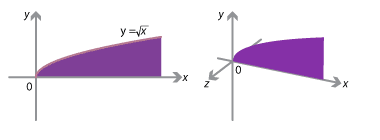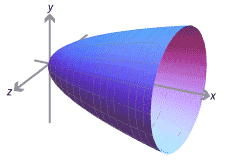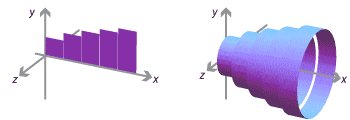### Integration and three dimensions

So far we have used integration to calculate areas, which are 2-dimensional. Integration can also be used to obtain 3-dimensional volumes.

For instance, take a graph $$y = f(x)$$ in the $$x–y$$ plane, and consider adding a third dimension: points in 3-dimensional space have coordinates $$(x,y,z)$$, and there is now also a $$z$$-axis. The figures below show the graph of $$y = \sqrt{x}$$, for $$0 \leq x \leq 5$$, in the plane and then adding a $$z$$-axis.Detailed description of diagrams

We can now consider rotating the curve around the $$x$$-axis. As we do, the curve sweeps out a surface, and the area under the curve sweeps out a 3-dimensional solid. The solid has rotational symmetry about the $$x$$-axis and is called a solid of revolution.We can estimate the area under the graph $$y=f(x)$$ in the plane by subdividing the $$x$$-interval and forming rectangles. Rotating these rectangles about the $$x$$-axis, we estimate the volume of the solid of revolution by cylinders.Detailed description of diagrams

Use our usual notation: we consider the graph of $$y=f(x)$$ between $$x=a$$ and $$x=b$$, and we divide the interval $$[a,b]$$ into $$n$$ subintervals $$[x_0, x_1]$$, $$\dots$$, $$[x_{n-1}, x_n]$$ of width $$\Delta x$$. As we have seen, for the right-endpoint estimate, the rectangles have width $$\Delta x$$ and height $$f(x_j)$$, and hence area $$f(x_j) \; \Delta x$$. Similarly, the cylinders have radius $$f(x_j)$$ and width $$\Delta x$$, and hence volume $$\pi \, f(x_j)^2 \, \Delta x$$. We can estimate the volume of the solid of revolution as $$\sum_{j=1}^n \pi \, f(x_j)^2 \, \Delta x$$. Taking the limit as $$n \to \infty$$, the volume of the solid of revolution is

$\int_a^b \pi \, f(x)^2 \; dx.$

More generally, if we have a solid bounded by the two parallel planes $$x=a$$ and $$x=b$$, and at each value of $$x$$ in $$[a,b]$$ the cross-sectional area of the solid is $$A(x)$$, then the volume of the solid is

$\int_a^b A(x) \; dx.$

Using this idea, it's possible to derive the formulas for the volumes of pyramids, prisms, and other solids. In higher mathematics courses one studies 3-dimensional (and higher-dimensional) volumes using multiple integrals.

Next page - Links forward - Arc length